• 模糊K均值算法python代码实现（FCM）
千次阅读 热门讨论
2018-01-08 09:58:15
# -*- coding:utf-8 -*-
from pylab import *
from numpy import*
import pandas as pd
import numpy as np
import operator
import math
import matplotlib.pyplot as plt
import random
#数据保存在.csv文件中
columns = list(df_full.columns)
features = columns[:len(columns)-1]
#class_labels = list(df_full[columns[-1]])
df = df_full[features]
# 维度
num_attr = len(df.columns) - 1
# 分类数
k =3
# 最大迭代数
MAX_ITER = 100
# 样本数
n = len(df)    #the number of row
# 模糊参数
m = 2.00

#初始化模糊矩阵
def initializeMembershipMatrix():
membership_mat = list()
for i in range(n):
random_num_list = [random.random() for i in range(k)]
summation = sum(random_num_list)
temp_list = [x/summation for x in random_num_list]#首先归一化
membership_mat.append(temp_list)
return membership_mat

#计算类中心点
def calculateClusterCenter(membership_mat):
cluster_mem_val = zip(*membership_mat)
cluster_centers = list()
cluster_mem_val_list = list(cluster_mem_val)
for j in range(k):
x=cluster_mem_val_list[j]
xraised = [e ** m for e in x]
denominator = sum(xraised)
temp_num = list()
for i in range(n):
data_point = list(df.iloc[i])
prod = [xraised[i] * val for val in data_point]
temp_num.append(prod)
numerator = map(sum, zip(*temp_num))
center = [z/denominator for z in numerator]#每一维都要计算。
cluster_centers.append(center)
return cluster_centers

#更新隶属度
def updateMembershipValue(membership_mat, cluster_centers):
#    p = float(2/(m-1))
data=[]
for i in range(n):
x = list(df.iloc[i])#取出文件中的每一行数据
data.append(x)
distances = [np.linalg.norm(list(map(operator.sub, x, cluster_centers[j]))) for j in range(k)]
for j in range(k):
den = sum([math.pow(float(distances[j]/distances[c]), 2) for c in range(k)])
membership_mat[i][j] = float(1/den)
return membership_mat,data

#得到聚类结果
def getClusters(membership_mat):
cluster_labels = list()
for i in range(n):
max_val, idx = max((val, idx) for (idx, val) in enumerate(membership_mat[i]))
cluster_labels.append(idx)
return cluster_labels

def fuzzyCMeansClustering():
# 主程序
membership_mat = initializeMembershipMatrix()
curr = 0
while curr <= MAX_ITER:#最大迭代次数
cluster_centers = calculateClusterCenter(membership_mat)
membership_mat,data = updateMembershipValue(membership_mat, cluster_centers)
cluster_labels = getClusters(membership_mat)
curr += 1
print(membership_mat)
return cluster_labels, cluster_centers,data,membership_mat

def xie_beni(membership_mat,center,data):
sum_cluster_distance=0
min_cluster_center_distance=inf
for i in range(k):
for j in range(n):
sum_cluster_distance=sum_cluster_distance + membership_mat[j][i]** 2 * sum(power(data[j,:]- center[i,:],2))#计算类一致性
for i in range(k-1):
for j in range(i+1,k):
cluster_center_distance=sum(power(center[i,:]-center[j,:],2))#计算类间距离
if cluster_center_distance<min_cluster_center_distance:
min_cluster_center_distance=cluster_center_distance
return sum_cluster_distance/(n*min_cluster_center_distance)
labels,centers,data,membership= fuzzyCMeansClustering()
print(labels)
print(centers)
center_array=array(centers)
label=array(labels)
datas=array(data)

#Xie-Beni聚类有效性
print("聚类有效性：",xie_beni(membership,center_array,datas))
xlim(0, 10)
ylim(0, 10)
#做散点图
f1 = plt.figure(1)
plt.scatter(datas[nonzero(label==0),0],datas[nonzero(label==0),1],marker='o',color='r',label='0',s=30)
plt.scatter(datas[nonzero(label==1),0],datas[nonzero(label==1),1],marker='+',color='b',label='1',s=30)
plt.scatter(datas[nonzero(label==2),0],datas[nonzero(label==2),1],marker='*',color='g',label='2',s=30)
plt.scatter(center_array[:,0],center_array[:,1],marker = 'x', color = 'm', s = 50)
plt.show()



#### 程序运行结果：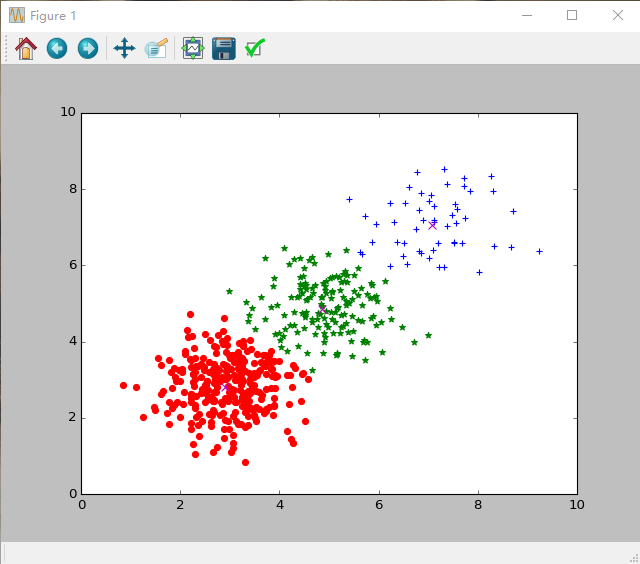更多相关内容
•人工智能
• 将模糊集理论和k-means聚类联系起来，设计了模糊k-means聚类算法，其聚类效果比单纯的k-means要好。
• 一．K-均值聚类（K-means）概述 1.聚类 “类”指的是具有相似形得几何。聚类是值将数据集划分为若干类，是的类内之间得数据最为相似，各类之间的数据相似度差别尽可能大。聚类分析就是以相似性为基础，对数据集进行...

一．K-均值聚类（K-means）概述

1.聚类

“类”指的是具有相似形得几何。聚类是值将数据集划分为若干类，是的类内之间得数据最为相似，各类之间的数据相似度差别尽可能大。聚类分析就是以相似性为基础，对数据集进行聚类划分，属于无监督学习。

2.无监督学习和监督学习

K-均值聚类属于无监督学习。监督学习知道从对象（数据）中学习什么，而无监督学习无需知道所要搜寻的目标，它根据算法得到数据的共同特征。比如用分类和聚类来说，分类事先就知道所要得到的类别，而聚类是以相似度为基础，将对象就分得不同的簇。

3.K-means

K-means算法是一种简单的迭代型聚类算法，采用距离作为相似性指标，从而发现给定数据集中的K个类，且每个类的中心是根据类中所有值的均值得到，每个类用聚类中心来描述。对于给定的一个包含n个d 维数据点的数据集X以及要分得的类别K，选取欧式距离作为相似度指标，聚类目标是使得各类的聚类平方和最小，即最小化：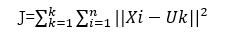结合最小二乘法和拉格朗日原理，聚类中心为对应类别中各数据点的平均值，同时为了使得算法收敛，在迭代过程中，应使最终聚类中心尽可能的不变。

二．K均值聚类算法实现

1.K均值聚类算法流程

K-means是一个反复迭代的过程，算法分为四个步骤：

1） 选取数据空间中的K个对象作为初始中心，每个对象代表一个聚类中心：

2） 对于样本中的数据对象，根据他们与这些聚类中心的欧氏距离，按距离最近的准则将它们分到距离它们最近的聚类中心（最相似）所对应的类；

3） 更新聚类中心：将每个类别中所有对象所对应的均值作为该类别的聚类中心，计算目标函数的值：

4） 判断聚类中心和目标函数的值是否发生改变，若不变，则输出结果，若改变，则返回2）。

2.K均值聚类Matlab实现

1）自主选择数据测试：利用mvnrnd（）函数在一定范围内创造随机数，并作图，生成的随机二维分布图形见图1：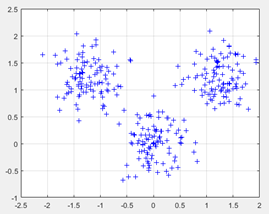2）分析类别数K的设定：K值的确定可以由以下方法确定：1、根据实际需要；2、肘部法则;3、轮廓系数;4、层次聚类;5、Canopy算法;6、间隔统计量 Gap Statistic;

本文采用层次聚类的方法确定K的取值。层次聚类是通过可视化然后认为去判断大致聚为积累，很明显在共同父节点的一颗子树可以被聚类为一类。分层聚类图见图2：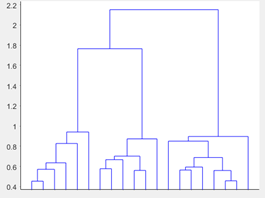图2

从图中我们可以看到K可以取3。

3）初始质心的选取：选择适当的初始质心是基本K-means算法的关键步骤。

方法1：随机选取初始质心，但是这样簇的质量常常很差。处理选取初始质心问题的一种常用技术是“多次运行，每次使用一组不同的随机初始质心，然后选取具有最小SSE的簇集。

方法2：取一个样本，并使用层次聚类技术对它聚类。从层次聚类中提取K个簇，并用这些簇的质心作为初始质心。适用于小样本。

方法3：随机选择第一个点，或去所有点的质心作为第一个点。然后，对于每个后继初始质心，选择已经选取过的初始质心最远的点。使用这种方法，确保了选择的初始质心不仅是随机的，而且是散开的。但是，这种方法可能选中离群点。

方法4：canopy算法。

本文随机选取质心，见图3：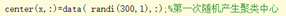图3

5） 距离的度量：常用的距离度量方法包括：欧几里得距离和余弦相似度。欧氏距离是最常见的距离度量，二余弦相似度是最常见的相似度度量，借助图4三维坐标系来看下欧氏距离和余弦相似度的区别：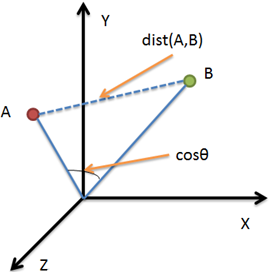图4：三维坐标系

从图上可以看出距离度量衡量的是空间各点间的绝对距离，跟各个点所在的位置坐标(即个体特征维度的数值)直接相关；而余弦相似度衡量的是空间向量的夹角，更加的是体现在方向上的差异，而不是位置。本文选择欧式距离来对距离进行度量，欧氏距离公式见（2）：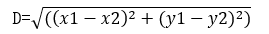6） 聚类效果展示如图5：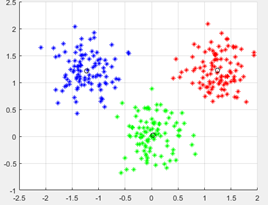图5

7） 聚类效果评估：Kmeans是一种非监督学习，没有标签和其他信息来比较聚类结果。从图5的结果可以清晰的看到算法具有一定的聚类效果，本文采用MCR进行验证，结果见图6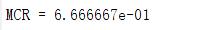图6

多次计算平均求得的MCR= 0.66,表明误分率还是蛮大的，聚类效果并不是很理想，究其原因：虽然算法收敛，但算法只是收敛到了局部最小值，而并非全局最小值。

三．模糊K均值算法

模糊K-均值算法由K-均值算法派生而来的。K-均值算法在聚类过程中，每次得到的结果虽然不一定是预期的效果，但类别之间的边界是明确的，聚类中心根据各类当前具有的样本进行修改。模糊K-均值算法在聚类过程中，每次得到的类别边界仍是模糊的，每类聚类中心的修改都需要用到所有样本，此外聚类准则也体现了模糊性。

四．模糊K-均值算法

1.模糊K-均值算法过程

模糊K-均值算法基本思想是首先设定一些类及每个样本对各类的隶属度；然后通过迭代，不断调整隶属度至收敛。收敛条件是隶属度的变化量小于规定的阈值。具体步骤如下：

（1）
确定模式类数K，1<K≤N，N是样本个数。

（2）
根据先验知识确定样本属于各类的隶属度，建立初始隶属度矩阵U（0）=[],其中i为类别编号、矩阵的行号，j为样本编号、矩阵的列号。表示第j个元素对第i个类的隶属度。对隶属度矩阵的第j列而言，它表示第j个元素分别对各模式类的隶属度，因此矩阵的每列元素之和为1。

（3）
求各类的聚类中心L是迭代次数。见公式（3）：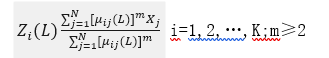式中，参数m≥2，是一个控制聚类结果模糊程度的参数。可以看出各聚类中心的计算必须用到全部的N个样本，这是与一般（非模糊）K-均值算法的区别之一。在一般（非模糊）K-均值算法中，某一类的聚类中心仅由该类样本决定，不涉及其他类。

（4）
计算新的隶属度矩阵U（K+1），矩阵元素计算如下：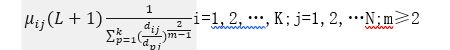式中，是第L次迭代完成时，第j个样本到第i类聚类中心的距离。为避免分母为零，特别的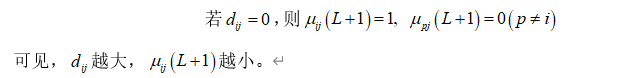5) 回到(3)求聚类中心，重复至收敛。收敛条件：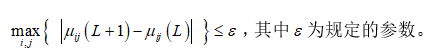，其中为规定的参数。 (6)

当算法收敛时，就得到了各类的聚类中心以及表示个样本对各类隶属程度的隶属度矩阵，模糊聚类到此结束。这时，准则函数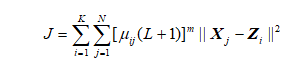达到最小。

(6) 根据隶属度矩阵U(L+1)进行聚类，按照隶属原则进行划分，即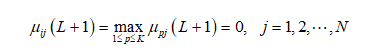.模糊K均值matlab实现

1．沿用上面用K均值聚类的的数据随机创造一组数据，见图6；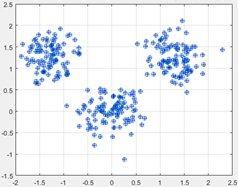图6

2.沿用上面K均值聚类确定K值的方法确定K值，取K=3。

3.根据先验知识确定初始隶属度矩阵，利用matlab的rand（）函数初始化隶属度矩阵U（0），并归一化，见图7：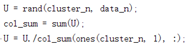图7

其中，cluster_n为聚类中心个数，即K值，data_n为样本点数，U为隶属度矩阵。

4．模糊K均值聚类时迭代的一步：计算新的隶属度矩阵，先求样本点到聚类中心的距离d，见图8：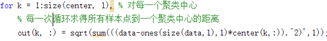图8

然后利用求得的距离d，带入公式求解新的隶属度矩阵，见图9：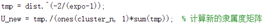图9

并求得目标函数值，见图10：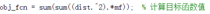图10

将新得的聚类中心和隶属度值重新分配进行下一次迭代。

5.设置隶属度最小变化量，当变化量小于这个阈值时，迭代终止，见图11：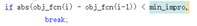图11

变化量小于设定阈值，跳出迭代。

3.聚类效果展示，见图12：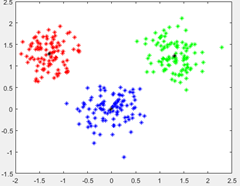图12

五．总结

通过实验，我们发现K均值聚类的优缺点：

优点：

1.算法快速、简单;

2.对大数据集有较高的效率并且是可伸缩性的;

3.时间复杂度近于线性，而且适合挖掘大规模数据集。

缺点：

1.在 K-means 算法中 K 是事先给定的，这个 K 值的选定是非常难以估计的。很多时候，事先并不知道给定的数据集应该分成多少个类别才最合适。

2.在 K-means 算法中，首先需要根据初始聚类中心来确定一个初始划分，然后对初始划分进行优化。这个初始聚类中心的选择对聚类结果有较大的影响，一旦初始值选择的不好，可能无法得到有效的聚类结果。

3.从 K-means 算法框架可以看出，该算法需要不断地进行样本分类调整，不断地计算调整后的新的聚类中心，因此当数据量非常大时，算法的时间开销是非常大的。

一K均值聚类程序：
clear all;close
all;clc;
%% 随机生成数据
% 第一组数据
mu1=[0 0 ]; %均值
S1=[.1 0 ;0.1]; %协方差
data1=mvnrnd(mu1,S1,100); %产生高斯分布数据
%第二组数据
mu2=[1.25 1.25 ];
S2=[.1 0 ;0 .1];
data2=mvnrnd(mu2,S2,100);
% 第三组数据
mu3=[-1.25 1.25 ];
S3=[.1 0 ;0 .1];
data3=mvnrnd(mu3,S3,100);
% 显示数据
plot(data1(:,1),data1(:,2),‘b+’);
hold on;
plot(data2(:,1),data2(:,2),‘r+’);
plot(data3(:,1),data3(:,2),‘g+’);
grid on;
% 三类数据合成一个不带标号的数据类
data=[data1;data2;data3];
%% 分层聚类确定K值
eucD =pdist(data,‘euclidean’);
cophenet(clustTreeEuc,eucD);
P3 = figure;clf;
[h,nodes] = dendrogram(clustTreeEuc,20);
set(gca,‘TickDir’,‘out’,‘TickLength’,[.0020],‘XTickLabel’,[]);
%% 随机产生聚类中心
N=3;%设置聚类数目
[m,n]=size(data);
pattern=zeros(m,n+1);
center=zeros(N,n);%初始化聚类中心
pattern(:,1:n)=data(:😅;
for x=1:N
center(x,:)=data( randi(300,1)😅;%第一次随机产生聚类中心
end
%% 迭代过程
while 1
distence=zeros(1,N);
num=zeros(1,N);
new_center=zeros(N,n);
for x=1:m
for y=1:N
distence(y)=norm(data(x,:)-center(y,:));%计算到每个类的距离
end
[~, temp]=min(distence);%求最小的距离
pattern(x,n+1)=temp;
end
k=0;
for y=1:N
for x=1:m
if pattern(x,n+1)== y
new_center(y,:)=new_center(y,:)+pattern(x,1:n);
num(y)=num(y)+1;
end
end
new_center(y,:)=new_center(y,:)/num(y);
if norm(new_center(y,:)-center(y,:))<0.1
k=k+1;
end
end
if k==N
break;
else
center=new_center;
end
end
[m,
n]=size(pattern);
%% 最后显示聚类后的数据
figure;
hold on;
for i=1:m
if pattern(i,n)==1
plot(pattern(i,1),pattern(i,2),‘r*’);
plot(center(1,1),center(1,2),‘ko’);
elseif pattern(i,n)==2
plot(pattern(i,1),pattern(i,2),‘g*’);
plot(center(2,1),center(2,2),‘ko’);
elseif pattern(i,n)==3
plot(pattern(i,1),pattern(i,2),‘b*’);
plot(center(3,1),center(3,2),‘ko’);
elseif pattern(i,n)==4
plot(pattern(i,1),pattern(i,2),‘y*’);
plot(center(4,1),center(4,2),‘ko’);
else
plot(pattern(i,1),pattern(i,2),‘m*’);
plot(center(4,1),center(4,2),‘ko’);
end
end
grid on;
[cidx3,cmeans3,sumd3,D3]= kmeans(data,3,‘dist’,‘sqEuclidean’);
P4 = figure;clf;
[silh3,h3] =silhouette(data,cidx3,‘sqeuclidean’);
%% 采用MCR判定聚类效果
B = pattern(:,3);
B = reshape(B,1,m);
A = [ones(1,100),2 * ones(1,100),3ones(1,100),4 * ones(1,100)];
sum = 0;
for i = 1:m
if ( A(1,i) ~= B(1,i))
sum = sum + 1;
end
end
MCR = sum / m;
fprintf(‘MCR =%d\n’,MCR);
二模糊K均值程序
function [U,
V,objFcn] = myfcm(data, c, T, m, epsm)
% fuzzy c-means
algorithm
% 输入： data： 待聚类数据，n行s列，n为数据个数，s为每个数据的特征数
% c ： 聚类中心个数
% m : 模糊系数
% 输出： U : 隶属度矩阵，c行n列，元素uij表示第j个数据隶属于第i类的程度
% V ： 聚类中心向量，c行s列，有c个中心，每个中心有s维特征
mydist.m myplot.m
if nargin < 3
T = 100;
%默认迭代次数为100
end
if nargin < 5
epsm = 1.0e-6; %默认收敛精度
end
if nargin < 4
m = 2;
%默认模糊系数值为2
end
[n, s] = size(data);
% 初始化隶属度矩阵U(0),并归一化
U0 = rand(c, n);
temp = sum(U0,1);
for i=1:n
U0(:,i) = U0(:,i)./temp(i);
end
iter = 0;
V(c,s) = 0; U(c,n) =
0; distance(c,n) = 0;
while( iter<T )
iter = iter + 1;
% U = U0;
% 更新V(t)
Um = U0.^m;
V = Um
data./(sum(Um,2)*ones(1,s)); % MATLAB矩阵相乘啊，好东西
% 更新U(t)
for i = 1:c
for j = 1:n
distance(i,j) =
mydist(data(j,:),V(i,:));
end
end
U=1/(distance.m.*(ones(c,1)*sum(distance.(-m))));
objFcn(iter) = sum(sum(Um.*distance.^2));
% FCM算法停止条件
if norm(U-U0,Inf)<epsm
break
end
U0=U;
end
myplot(U,objFcn);
function d = mydist(X,Y)
% 计算向量Y到向量X的欧氏距离的开方
d =
sqrt(sum((X-Y).^2));
end

展开全文聚类 深度学习
• 该程序说明了图像的模糊 c 均值分割。 该程序使用模糊 k 均值算法将输入图像转换为两段。 输出在当前目录中存储为“fuzzysegmented.jpg”。通过稍微修改给定的代码，该程序可以概括为从图像中获取“n”个片段。matlab
• 解决神经网络算法中样本数据包含大量与目标数据无关的属性而导致网络训练时间长、效率低的问题，提出基于改进模糊k均值(FKM)和BP神经网络算法的数据挖掘模型．利用改进的FKM聚类算法对输入数据的属性进行聚类，摈弃...
• 1.4 模糊K均值算法伪代码流程 2. 算法相关问题 2.1 质心定义问题 2.2 聚类效果评估方法 （1）轮廓系数 轮廓系数（Silhouette Coefficient），是聚类效果好坏的一种评价方式。它结合了凝聚度和分离度两...

这篇文章是根据作业修改后得到的，个人感觉写的比较详细了。但还有许多不足，希望大家评论指出。

## K均值聚类与模糊K均值

### 1. 算法原理及流程

相关名词解释如表1。
表1-相关名词解释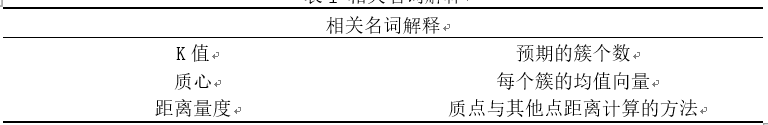##### 1.1 K均值算法原理

K均值是基于原型的、划分的聚类计数。K均值使用质心定义原型，质心是一组点的均值。
1）首先，选择K个初始质心（通常随机初始化），其中K是用户预期的簇个数。
2）每个点指派到最近的质心，而指派到一个质心的点集合为一个簇。这里的距离量度有多种，通常采用欧几里得距离：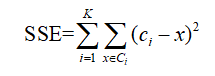SSE为误差的平方和（Sum of Squared Error, SSE）， 为第 个簇， 为 中的点， 为第 个簇的均值。
3）然后，根据指派到簇的点，更新每个簇的质心。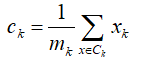4）重复指派和更新步骤，直到簇不发生变化，或等价为质心不发生变化。

##### 1.2 K均值算法伪代码流程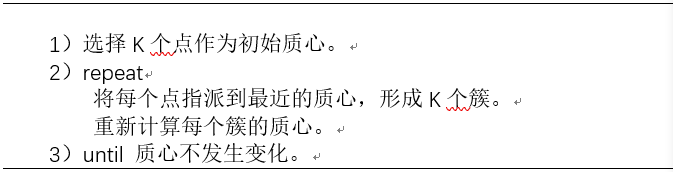##### 1.3 模糊K均值算法原理

在大部分情况下，数据集中的对象并不能划分为明显分离的簇，且指派一个对象到一个特定的簇也有一定的任意性。此时采用模糊的概念，对每个对象和每个簇赋予一个权值，指明该对象属于该簇的程度。这样可以更好的对该点进行划分。##### 1.4 模糊K均值算法伪代码流程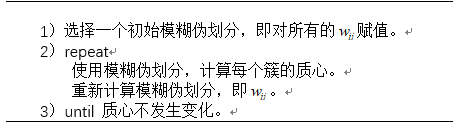### 2. 算法相关问题

##### 2.1 质心定义问题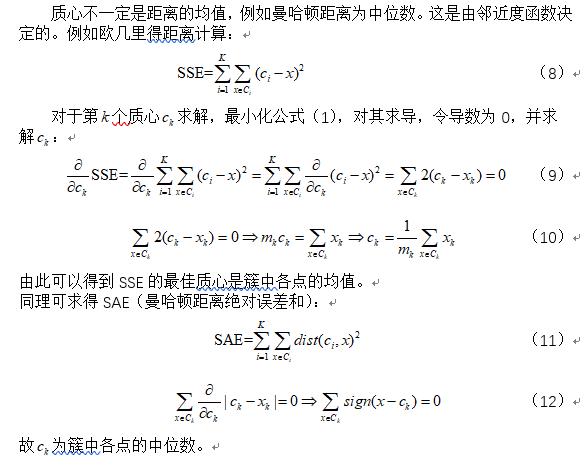##### 2.2 聚类效果评估方法

（1）轮廓系数
轮廓系数（Silhouette Coefficient），是聚类效果好坏的一种评价方式。它结合了凝聚度和分离度两种因素。可以用来在相同原始数据的基础上用来评价不同算法、或者算法不同运行方式对聚类结果所产生的影响。

轮廓系数的值处于-1~1之间，值越大，表示聚类效果越好。具体计算方法如下：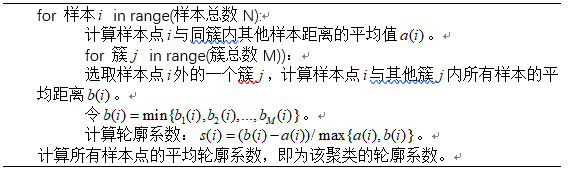（2）手肘法
手肘法利用SSE曲线判别最佳簇数K，SSE公式在上面已给出。通过对不同簇K进行统计，可以得到手肘样的曲线，在肘处的SSE较小，且随着K的增加，SSE逐渐趋于平稳。
（3）相似度矩阵
相似度矩阵按照簇标号调整数据对象距离矩阵的行列次序，并将数据对象间的距离转换成相似度。明显分离的簇会在会显示很强的块对角模式，可以较形象的反应聚类效果。但是对于大型数据集，该方法开销较大，复杂度为O（n2）。

注：不同距离量度下的聚类效果本应采用相似度矩阵等宏观检测，但是由于时间关系仅采用了SSE与轮廓系数来一定程度上反应聚类效果，SSE与轮廓系数中的距离量度均统一为欧式距离。

### 3. K均值仿真测试

本文代码在python3.7环境下运行，数据集自定义在.txt文件中。

###### 3.1 不同簇数量K下的仿真

初始质心的选取采用随机数生成的方式，计算输入数据集x与y维的最小值与最大值，然后计算两者的范围。则每个质心的x与y维值为最小值加上范围之间的某个值。距离度量统一采用欧式距离，分类结果的评价采用手肘法与轮廓系数结合的方法进行评价。从簇中可以看到存在较小簇时，不容易让其单独分出来（簇K=5），个人感觉K=4合K=6时的分类效果较好，K=4时能够将较大的簇单独分类处理，K=6时能够将小的簇分类出来，但是有较大的簇被分为两类。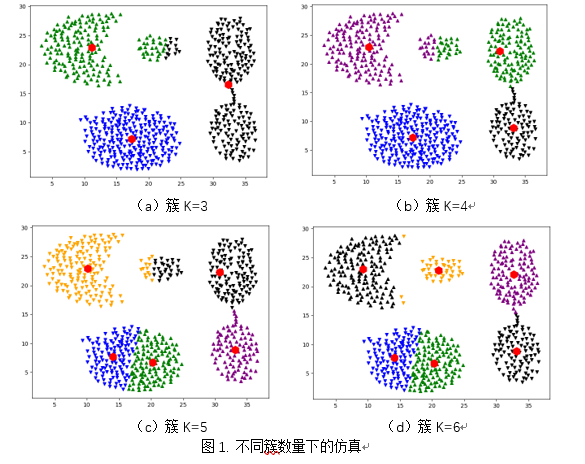从图中曲线可以较为明显看出较高的轮廓系数出现在K=4时，第2个峰值出现在K=6时。而对于SSE曲线则呈不断衰减的趋势，到K=6以后衰减速率较慢。因此综合来看，簇K=6时的分类效果较好。

##### 3.2 不同距离量度下的仿真

除了欧式距离，这里再采用三种其他距离作为对比。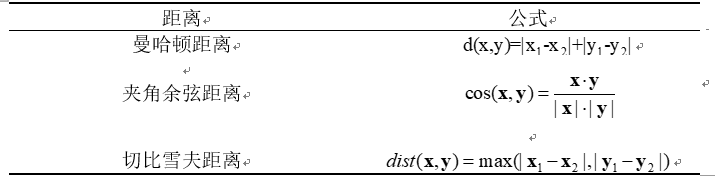其中对于曼哈顿距离要对质心的选取要采用中位数，而不是取均值。这里分析了四种不同的距离量度，初始质心的选取与3.1一致，簇K按照数据分布分为5簇数据。对分类好的数据分别拿出来对比了一下，并记录了每种距离量度下的SSE与轮廓系数，然后进行了对比。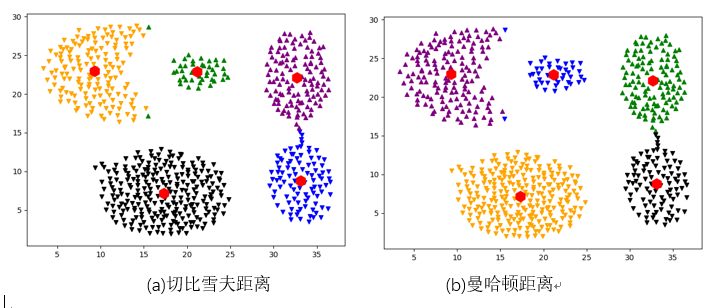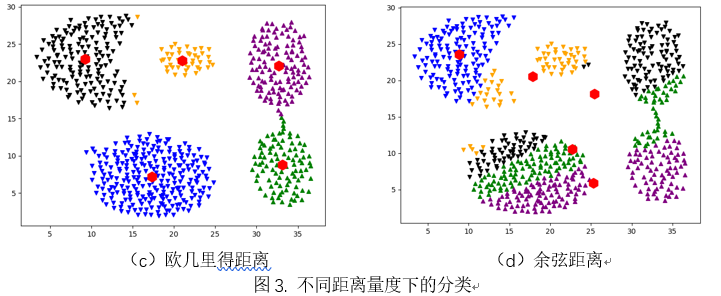从图3中可以看出前三种距离量度分类较好，但是对于余弦距离来说，分类结果比较不理想。但是再看下SSE与轮廓系数曲线。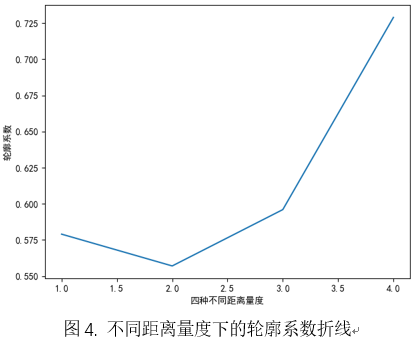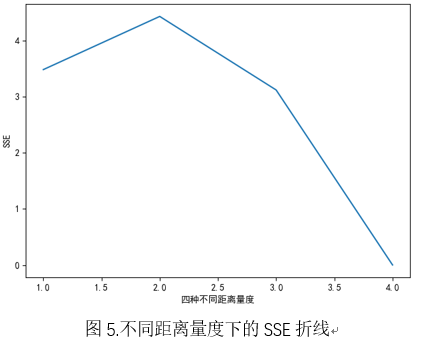图中1，2，3，4分别对应欧几里得距离、曼哈顿距离、切比雪夫距离和余弦距离。从图中可以看出曼哈顿距离轮廓系数最小，且SSE最高，说明该方法对这种数据的距离效果不佳。而欧几里得与切比雪夫的轮廓系数与SSE相差无几，对于余弦距离的数据就有点夸张了，轮廓系数有0.729，而SSE有0.003，但从肉眼观测来看该分类并不是很好。因为余弦距离是两向量之间的角度余弦值，故这种数据的分类其实并不适合。

##### 3.3 不同初始质心选取条件下的仿真

原来是采用范围内取随机数的方法进行指派初始质心，这样的效果有时较好有时较差，并不是特别稳定。

层次聚类是另一种指派质心的方式，通过层次聚类，划分k个层次，计算出每个簇对应的质心作为K-Means算法的初始质心。这种方法可以很好地解决初始质心指派不合理的问题。但是也有局限性。

K-Means++方法中初始质心的选取也是一种较新的方式，第一个质心是随机选择的，接下来的质心基于样本点与最近质心的距离，距离越大越可能被选为下一个质心，直到选择完k个质心。该方法有效地解决了关于初始质心的选取问题，目前已经成为了一种硬聚类算法的标准。但是该方法无法解决离群点问题。

这里仅采用了K-Means++中初始质心选取的方法作为对比，在K=5条件下，采用欧几里得距离度量，并统计轮廓系数，K-Means++普遍比随机质心的轮廓系数高。

### 4. 模糊K均值仿真测试

在原来函数体的框架下新建了新的函数，改变了质心更新公式、SSE公式，并添加了每个点对不同簇的权值计算公式。在与上面仿真类似的情况下，对模糊K均值进行了同样的仿真。

##### 4.1 不同簇数量K下的仿真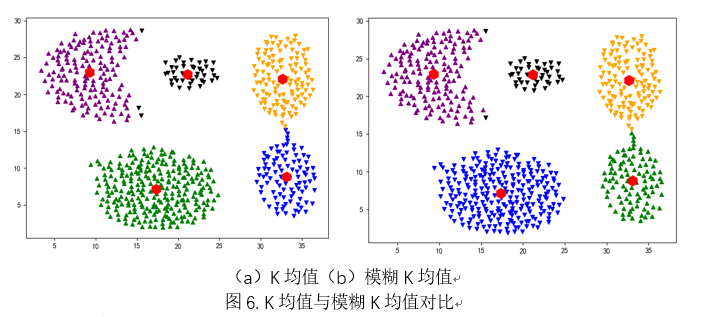这里并没有一一列举不同簇数量K下的仿真图，其效果与K均值类似。这里将K均值与模糊K均值在K=5的情况下进行了仿真对比，其实效果相差不大。但是模糊K均值较多出现将下面的那个大簇分为两类，而较少识别出较小的簇，这样来看这个对比其实效果不大。再看一下SSE曲线与轮廓曲线，SSE曲线与K均值的类似，也是在K=6时开始趋向平稳，SSE较低的原因是因为每个点与质心的距离乘了权值的缘故。再看轮廓系数曲线，与K均值在K=6时明显不同，且呈不断降低的趋势，整体数值相差不大。K取4或5轮廓系数会更好。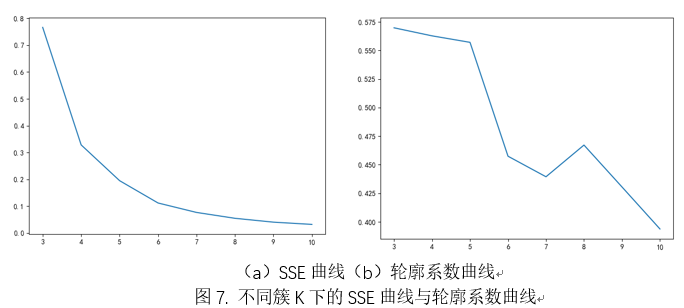##### 4.2不同距离量度下的仿真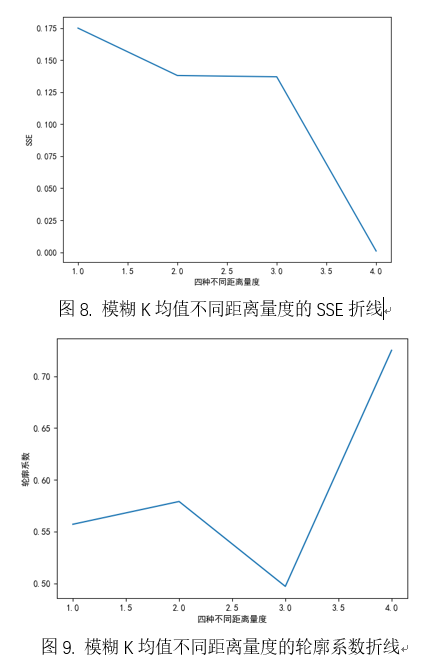从图中可以看出余弦度量还是与K均值一样，轮廓系数极高，SSE极低，但是分类效果极差，因为它本身不适合这种数据的聚类。而欧几里得的轮廓系数是与其他两个相比较高的距离量度，且SSE与曼哈顿相同，故在模糊K均值中采用欧几里得距离更好。

### 5. 小结

K均值简单并可以用于各种数据类型。然而，K均值并不适合所有的数据类型。它不能处理非球形簇、不同尺寸和不同密度的簇，尽管指定足够大的簇个数K时它通常可以发现纯子簇。对包含离群点的数据进行聚类时，K均值也有问题。在这种情况下，离群点检测与删除大有帮助。最后，K均值仅限于具有质心概念的数据。

本次主要对比了K均值与模糊K均值在不同簇数K的情况下、不同距离量度下和不同初始质心选取的情况下的分类效果，采用了SSE和轮廓系数评价聚类效果。对于不同的数据类型应选取适合的距离量度，再根据数据大致分类缺点簇K的大小，初始质心选取可以采用与K-Means++中的方法，这样可以获得较稳定质量较高的聚类效果。

### 参考文献

 数据库https://scikit-learn.org/stable/datasets/index.html#iris-plants-database
 Pang-Ning Tan, Michael Steinbach, Vipin Kumar, 数据挖掘导论,2011
 各种距离度量https://blog.csdn.net/lj6052317/article/details/78770383

### 代码

from numpy import *
import numpy as np
import matplotlib.pyplot as plt
import operator
import random
from scipy.spatial.distance import pdist
from pylab import *
# 正常显示中文
mpl.rcParams['font.sans-serif'] = ['SimHei']
plt.rcParams['axes.unicode_minus'] = False
INF = 9999999.0
'''
加载数据集
:param fileName:
:return:
'''
# 初始化一个空列表
dataSet = []
# 读取文件
fr = open(fileName)
# 循环遍历文件所有行
# 切割每一行的数据
curLine = line.strip(',').split(',')
fltLine = list(map(float, curLine))    # 映射所有的元素为 float（浮点数）类型
dataSet.append(fltLine)
return dataSet
def distEclud(vecA, vecB):
"""欧式距离
输入：向量A, 向量B
输出：两个向量的欧式距离
"""
a = (vecA[0, 0] - vecB[0, 0]) ** 2
b = (vecA[0, 1] - vecB[0, 1]) ** 2
return sqrt(a+b)
def distManhattan(vecA, vecB):
'''曼哈顿距离
输入：向量A, 向量B
输出：两个向量的曼哈顿距离
'''
return np.sum(np.abs(vecA-vecB))
def distCosine(vecA, vecB):
'''余弦距离
输入：向量A, 向量B
输出：两个向量的余弦距离
'''
return pdist(np.vstack([vecA, vecB]), 'cosine')
def distChebyshev(vecA, vecB):
'''切比雪夫距离
输入：向量A, 向量B
输出：两个向量的切比雪夫距离
'''
return np.max(np.abs(vecA-vecB))
def randCent(dataSet, k):
"""生成随机质心
输入：数据集, 聚类个数
输出：k个随机质心的矩阵
"""
n = dataSet.shape #每个数据的维度
centroids = mat(zeros((k, n))) # 生成k*n维数据
for j in range(n):
minJ = min(dataSet[:, j]) # 第j列最小值
rangeJ = float(max(dataSet[:, j]) - minJ) # 第j列最大值与最小值的差值
centroids[:, j] = minJ + rangeJ * np.random.rand(k, 1) # 最小值加上差值的（0，1）之间的倍数
return centroids
def w_update(dataSet, centroids, clusterAssment, distMeans=distEclud):
"""更新权重值
输入：数据集, 质心
输出：更新后的权值
"""
for j in range(dataSet.shape):
dist_all = 0
for cen in range(len(centroids)):
dist = distMeans(dataSet[j, :], centroids[cen, :])
dist_all += dist
for cen in range(len(centroids)):
dist_self = distMeans(dataSet[j, :], centroids[cen, :])
clusterAssment[j, cen+2] = dist_self/dist_all
return clusterAssment
def kMeans(dataSet, k, distMeans=distEclud, createCent=randCent):
"""
输入：数据集, 聚类个数, 距离计算函数, 生成随机质心函数
输出：质心矩阵, 簇分配和距离矩阵
"""
m = dataSet.shape
clusterAssment = mat(zeros((m, 2))) # 初始化聚类矩阵
centroids = createCent(dataSet, k) # 生成随机质心
clusterChanged = True #启动
while clusterChanged:
clusterChanged = False
for i in range(m): # 寻找最近的质心
minDist = INF
minIndex = -1
for j in range(k):
distJI = distMeans(centroids[j, :], dataSet[i, 0:2]) #距离方法,计算该点与质心的距离
if distJI < minDist:   # 得到与质心距离最少的距离下标
minDist = distJI
minIndex = j
if clusterAssment[i, 0] != minIndex: # 只要有一个点的簇发生变化，就继续进行
clusterChanged = True
clusterAssment[i, :] = minIndex, minDist #存储该点的簇类，该点到簇心的距离
for cent in range(k): # 更新质心的位置
ptsInClust = dataSet[nonzero(clusterAssment[:, 0].A == cent)]
centroids[cent, :] = mean(ptsInClust, axis=0) #计算均值
return centroids, clusterAssment
def fuzzy_kmeans(dataSet, k, distMeans=distEclud, createCent=randCent):
"""
输入：数据集, 聚类个数, 距离计算函数, 生成随机质心函数
输出：质心矩阵, 簇分配和距离矩阵
"""
m = dataSet.shape
clusterAssment = mat(zeros((m, k+2)))  # 初始化聚类矩阵
centroids = createCent(dataSet, k)  # 生成随机质心
clusterChanged = True  # 启动
clusterAssment[:, 2:k+2] = 1/k
while clusterChanged:
clusterChanged = False
for i in range(m):  # 寻找最近的质心
minDist = INF
minIndex = -1
for j in range(k):
distJI = (clusterAssment[i, j+2]**2)*distMeans(centroids[j, :], dataSet[i, 0:2])  # 距离方法,计算该点与质心的距离
if distJI < minDist:  # 得到与质心距离最少的距离下标
minDist = distJI
minIndex = j
if clusterAssment[i, 0] != minIndex:  # 只要有一个点的簇发生变化，就继续进行
clusterChanged = True
clusterAssment[i, 0:2] = minIndex, minDist  # 存储该点的簇类，该点到簇心的距离
# 更新权值
clusterAssment = w_update(dataSet[i, :], centroids, clusterAssment)
c = mat(zeros((3, k)))
for cent in range(dataSet.shape): #更新质心的位置
c[2, int(clusterAssment[cent, 0])] += clusterAssment[cent, int(clusterAssment[cent, 0])+2]
c[0, int(clusterAssment[cent, 0])] += clusterAssment[cent, int(clusterAssment[cent, 0])+2]*dataSet[cent, 0]
c[1, int(clusterAssment[cent, 0])] += clusterAssment[cent, int(clusterAssment[cent, 0])+2]*dataSet[cent, 1]
for cent in range(k):
cc0 = c[0, cent]/c[2, cent]
cc1 = c[1, cent]/c[2, cent]
centroids[cent, :] = cc0, cc1  # 计算均值
return centroids, clusterAssment
def plotFeature(dataSet, centroids, clusterAssment):
m = shape(centroids)
fig = plt.figure()
scatterMarkers = ['v', '^']
scatterColors = ['blue', 'green', 'black', 'purple', 'orange', 'black', 'yellow']
for i in range(m):
ptsInCurCluster = dataSet[nonzero(clusterAssment[:, 0].A == i), :]
markerStyle = scatterMarkers[i % len(scatterMarkers)] # 选择标记点
colorSytle = scatterColors[i % len(scatterColors)] # 选择颜色
# flatten为返回一维数组
ax.scatter(ptsInCurCluster[:, 0].flatten().A, ptsInCurCluster[:, 1].flatten().A, marker=markerStyle, c=colorSytle, s=30) # 添加每类数据点
ax.scatter(centroids[:, 0].flatten().A, centroids[:, 1].flatten().A, marker='h', c='red', s=200) # 添加质心的位置
def silhouette(dataset, clustAssing, K, distMeans=distEclud):
'''计算每个点的轮廓系数
:param dataset:所有数据集
:param clustAssing:输入为每个簇的标签和其到簇心的距离
:return:返回平均轮廓系数，和数组
:k:簇数量
'''
mean_s = 0
clustAssing_new = mat(zeros((dataset.shape, 2)))
for i in range(dataset.shape):
a = 0 ; n1 = 0
buffer = []
for k in range(K):
buffer.append([])
for j in range(dataset.shape):
if i == j :
continue
elif clustAssing[i, 0] == clustAssing[j, 0]: #计算同簇内的距离
n1 += 1
distJI = distMeans(dataset[j, 0:2], dataset[i, 0:2])  # 距离方法,计算该点与质心的距离
a += distJI
elif clustAssing[i, 0] != clustAssing[j, 0]: #计算不同簇内的距离
distJI = distMeans(dataset[j, 0:2], dataset[i, 0:2])
buffer[int(clustAssing[j, 0])].append(distJI)
b_min = INF
for p in range(K):
if p == clustAssing[i, 0]:
continue
else:
b_buff = 0
for q in range(len(buffer[p])):
b_buff += buffer[p][q]
try:
b_buff = np.round(b_buff / len(buffer[p]), 5)
except Exception as e:
print(e)
if b_buff <= b_min:
b_min = b_buff
aa = np.round(a/n1, 5)
s = (b_min - aa)/max(aa, b_min)
clustAssing_new[i, :] = clustAssing[i, 0], s
mean_s += s
mean_s = mean_s/dataset.shape
return mean_s, clustAssing
def SSE(K, clustAssing):
value = []
SSE_mean = 0
for k in range(K):
value.append([])
for i in range(clustAssing.shape):
value[int(clustAssing[i, 0])].append(clustAssing[i, 1])
for k in range(K):
SSE_mean += np.mean(value[k])
SSE_mean /= K
return SSE_mean
def main():
dataSet = mat(dataset)
resultCentroids, clustAssing = fuzzy_kmeans(dataSet, 5)
#  resultCentroids, clustAssing = kMeans(dataSet, 5)
plotFeature(dataSet, resultCentroids, clustAssing)
mean_s, clustAssing_new = silhouette(dataSet, clustAssing, 5)
mean_sse = SSE(5, clustAssing)
print(mean_s, mean_sse)
plt.show()
#   plt.xlabel('四种不同距离量度')  # 将坐标系x轴命名为x1
#   plt.ylabel('轮廓系数')  # 将坐标系y轴命名为y1
#   plt.plot([1,2,3,4],[0.557,0.579,0.497,0.725])
#   plt.show()
#    performence = [[], []]
#    for i in range(3, 11):
#        resultCentroids, clustAssing = fuzzy_kmeans(dataSet, i)
# #      print(i, resultCentroids)
#        mean_s, clustAssing_new = silhouette(dataSet, clustAssing, i)
#         # mean_s = SSE(i, clustAssing)
#        # print('簇K%-2d的SSE为 %.5f'%(i, mean_s))
#        print('簇K %-2d 的轮廓系数为 %.5f'%(i, mean_s))
#        performence.append(i)
#        performence.append(mean_s)
#    plt.plot(performence, performence)
#    plt.show()
if __name__ == '__main__':
main()


### 如果觉得写得不错，请点赞、关注、评论。非常感谢~~

展开全文python 聚类
• 模糊 k-means 聚类算法的核心思想在于最小化式(下式)所示的目标函数： 其中，µij表示矢量 xj被分到第i类的概率，µ=[µij]是一个K×T的矩阵，满足下面的一些 性质： 同时，m(m≥2)是控制模糊度的一个加权指数...

假设特征矢量集，X={x1,x2,...,xT}，T 是语音帧数，每个矢量 xj 都是 维的，代表的是第 j 帧的特征参数。模糊 k-means 聚类算法的核心思想在于最小化式(下式)所示的目标函数：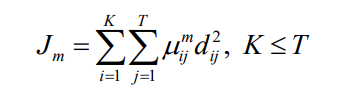其中，µij 表示矢量 xj 被分到第 i 类的概率，µ=[µij]是一个 K×T 的矩阵，满足下面的一些 性质：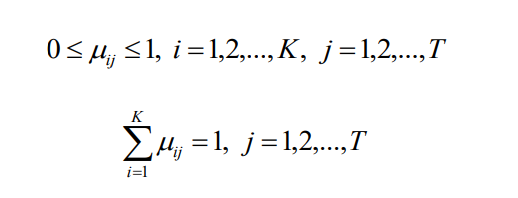同时，m(m≥2)是控制模糊度的一个加权指数，dij2 表示的是 xj 与 ci 之间的距离，定义式 如下所示：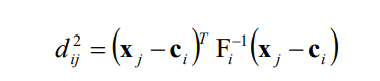其中，ci 指的是第 i 个聚类中心，Fi 表示的是第 i 个聚类的模糊协方差矩阵，通过令 Jm 相对 µ和 c 的梯度为 0 求得，如下式：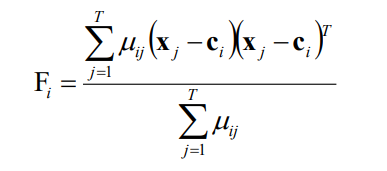只有在满足下面两式的情况下，才能求得目标函数(即本文第一个公式)的最小化。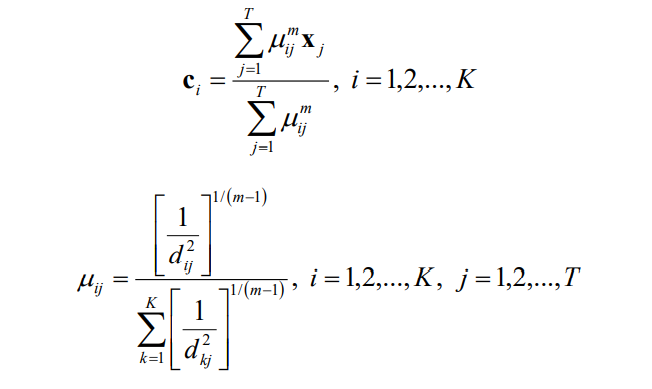上面两个式子的求解过程如下：

(1) 设定聚类数目 K，加权指数 m(m≥2)，终止条件 ε(ε>0)以及迭代次数 l。

(2) 初始化隶属度矩阵 µ(0)。

(3) 计算聚类中心 ci (l)。

(4) 更隶属度矩阵 µij (l)，直到满足||µ (l) -µ (l-1)||<ε 或是达到最大迭代次数。

本文中的最终特征矢量的分类就是用到了最大隶属度值，也就是说，对于任意的一个特 征矢量 xj(j=1,2,....,T)，如果 µij=max{µ1j,µ2j,...,µKj}成立，那么 xj 就属于第 i 类。

本文中总的高斯混合分量 M 是 64 个，每一类里高斯混合分量的个数 Mi 是由该类中训练 特征矢量的帧数 Ti 占总的训练特征矢量帧数 Tall 的比例来决定的，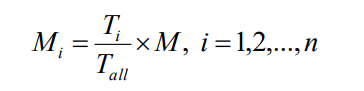其中，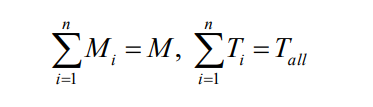n 是聚类数。

给定 I 和 Ii 分别表示总的训练迭代次数和每个聚类的训练迭代次数。因此，GMM 模型参 数的估计所需的计算时间 P 如下式所示：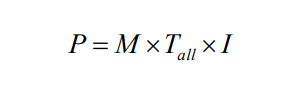聚类之后，上式可表示为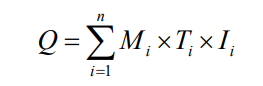假定每个聚类中的高斯混合分量个数是相同的，则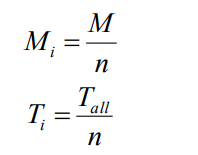将上面两个公式带入式(聚类之后下面的公式)可得：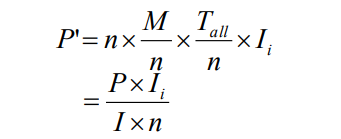由该式可知，随着聚类的每一类中的训练矢量的减少，以及每一类的训练矢量主要集中 在聚类中心周围，训练速度可以得到一定程度的提高。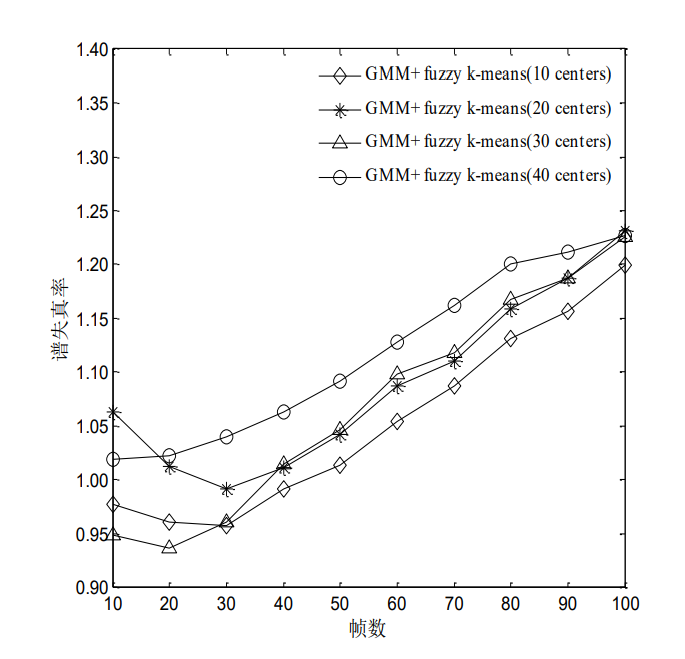上图显示的基于不同聚类中心的模糊 K-means 聚类的频谱失真，聚类中心数目分别是 10，20，30 和 40，从图中可以很明显的看出，随着聚类中心数目的增加，频谱失真也随之变 大，因此，本文采用的聚类中心数目为 10 个。

可以关注音频核公众号了解更多哦展开全文机器学习 语音识别
• ## 模糊K均值聚类算法

千次阅读 2019-04-14 21:08:06
模糊K均值聚类并不是将对象分给最近的簇，而是计算向量和各个簇之间的相关性。假设有一个向量V，有K个簇，V和这K个簇的中心的距离是d1,d2,....,dkd_1,d_2,....,d_kd1​,d2​,....,dk​,则V到第一个簇的相关性 U1=1d...机器学习 k均值 人工智能
• 该程序可以实现MATLAB中模糊K均值算法的实现，计算结果为聚类中心以及每个样本对聚类中心的隶属度函数
• 模糊Kmeans算法，java语言编写，里面的py文件是对聚类结果可视化。
• 老师，我用您书中的程序对12年4月房价指数做了k均值聚类和模糊c均值聚类，k均值的结果是ans ='北京''天津''石 家 庄''太原''呼和浩特''沈阳''大连''长春''哈 尔 滨''上海''南京''合肥''福州''厦门''南昌''济南''郑州...
• 文件详细介绍了模糊k均值算法的过程，并举例实现，然后给出了matlab实现的详细代码。
• 非监督分类——k均值算法 图像分类的概念：将图像中的像素点划分为不同的类别，每个类别具有特定的物理含义。例如不同的地物类型，包括草地、水泥地、建筑、道路、森林、山地等。 图像分类的两个步骤：特征提取与...matlab 图像识别
• 最近学模式识别其中一个算法模糊K均值算法我用C++实现的，我也是刚刚学习，算法用C++写的，写的很简陋还请多包涵，发上来共享一下，给有需要的网友看看吧，基于VC++的控制台程序。。
• 基于模糊K均值FuzzyKMeans聚类的协同过滤推荐算法代码实现（输出聚类计算过程，分布图展示） 聚类(Clustering)就是将数据对象分组成为多个类或者簇 (Cluster)，它的目标是：在同一个簇中的对象之间具有较高的相似度...
• 本文主要介绍K-means和FCM聚类算法的区别与应用机器学习 python
• 数据收集数据问题背景数据来源数据描述2.K-均值2.1K-均值原理2.2K-均值分析2.3总结3.C-均值3.1C-均值原理3.2C-均值分析3.3总结4.两种聚类对比（分类结果，优点，缺点） 1.数据收集 数据问题背景 数据来源 数据...
• 模糊c均值算法matlab程序时间：2019-12-21模糊c均值算法matlab程序相关问题:匿名网友:function [center,U,obj_fcn] = FCMClust(data,cluster_n,options)% FCMClust.m 采用模糊C均值对数据集data聚为cluster_n类%% ...
• K-均值K-means）算法是一种聚类算法k是用户指定的簇个数，将相似数据点归于同一簇，不相似数据点归于不同簇。机器学习
• 在分析归纳原有聚类方法不足的基础上，结合粗糙理论和模糊理论，给出了改进的粗糙模糊K-均值聚类算法；设计了新的模糊粗糙K-均值聚类算法，并验证了该聚类算法的有效性；进而将这两种聚类算法应用到支持向量机中，对...
• 首先,Sink节点收集各子区域的节点位置信息,并行运行模糊K均值算法将网络区域分为若干大小规模不同的簇,并将数据中心拟合到初始簇头节点。然后,以最大化节点剩余能量和最小化节点与簇头以及簇头与Sink节点的距离为...
•研究论文
• function [center, U, obj_fcn] = FCMClust(data, cluster_n, options)% FCMClust.m 采用模糊C均值对数据集data聚为cluster_n类%% 用法：% 1. [center,U,obj_fcn] = FCMClust(Data,N_cluster,options);% 2. [center,...
• 最近更新文章的频率太低了，主要原因是不想为了发文章而发文章，想潜心研究，写好文章，顺便想说一句开源万岁，最近一个月虽然一直在研究脑电信号特征提取和分类的算法，虽然待在实验室的时间不短，但是效率很低，...
• 模糊c均值算法FCM分类遥感12-2班刘杰1204090215FCM聚类算法模糊c-均值聚类算法 fuzzy c-means algorithm (FCMA)或称 FCM在众多模糊聚类算法中模糊C-均值 FCM 算法应用最广泛且较成功它通过优化目标函数得到每个样本......